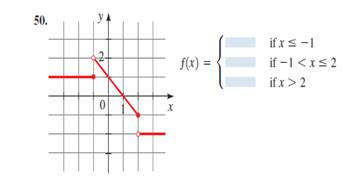# The formula of the curve.### Precalculus: Mathematics for Calcu...

6th Edition
Stewart + 5 others
Publisher: Cengage Learning
ISBN: 9780840068071### Precalculus: Mathematics for Calcu...

6th Edition
Stewart + 5 others
Publisher: Cengage Learning
ISBN: 9780840068071

#### Solutions

Chapter 2.2, Problem 50E
To determine

## To find : The formula of the curve.

Expert Solution

f(x)={2     if  x<2x       if 2x22       if x>2

### Explanation of Solution

Given information :Formula used :

The equation of the straight line parallel to X-axis can be written as y=a , where a is the Y-coordinate of any point lie on that line.

The equation of the straight line can be written as y=mx+c , where m is the gradient of the line and c is the Y intercept.

Calculation :

The graph right side of the x=2 is a line parallel to X-axis.

It is clear that all the Y coordinates of the points lie on that line is (2) . Therefore, the equation of the that line is y=2

The graph left side of the x=1 is a line parallel to X-axis.

It is clear that all the Y coordinates of the points lie on that line is 1 . Therefore, the equation of the that line is y=1 .

The graph between x=1 and x=2 is a straight line .

It cut the Y-axis at (0,1) . Hence, c=1

m=1

Therefore, the formula of the curve is y=x+1

If we combine three equation and take y=f(x) , it can be written as

f(x)={1               if  x1x+1       if 1<x22            if x>2

### Have a homework question?

Subscribe to bartleby learn! Ask subject matter experts 30 homework questions each month. Plus, you’ll have access to millions of step-by-step textbook answers!